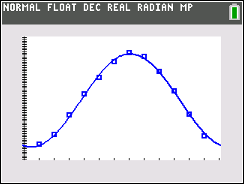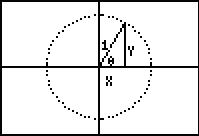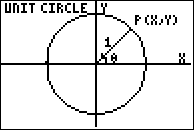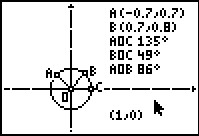### Trig Proofs

Students perform trigonometric proofs and verifying each proof through graphing.
•TI-84 Plus CE
•TI-84 Plus C Silver Edition
• TI-84 Plus Silver Edition
• TI-84 Plus### Trig Ratios - IB

In this activity, students will use Cabri™ Jr. to discover the relationship between the trigonometric functions: sine, cosine and tangent and the side length ratios of a right triangle.

•TI-84 Plus CE
•TI-84 Plus C Silver Edition
• TI-84 Plus Silver Edition
• TI-84 Plus### Find That Sine - IB

Sinusoidal regression is used to determine equations to model various data sets and the equations are used to make inferences.
•TI-84 Plus CE
•TI-84 Plus C Silver Edition
• TI-84 Plus Silver Edition
• TI-84 Plus### Trigonometric Identities

Students will verify, prove, and explore trigonometric identities symbolically, numerically, and graphically.
•TI-84 Plus CE
•TI-84 Plus C Silver Edition
• TI-84 Plus Silver Edition
• TI-84 Plus### Trigonometric Patterns

Students use the unit circle to examine patterns in the six trigonometric functions.
•TI-84 Plus CE
•TI-84 Plus C Silver Edition
• TI-84 Plus Silver Edition
• TI-84 Plus### Unit Circle

Students discover the relationship between the trigonometric functions sine, cosine, and tangent and the side length ratios of a right triangle.
•TI-84 Plus CE
•TI-84 Plus C Silver Edition
• TI-84 Plus Silver Edition
• TI-84 Plus### Vertical and Phase Shifts

Students explore vertical and phase shifts of sine and cosine functions.
•TI-84 Plus CE
•TI-84 Plus C Silver Edition
• TI-84 Plus Silver Edition
• TI-84 Plus••